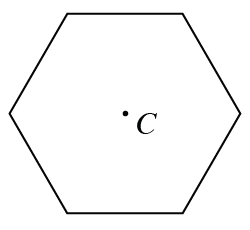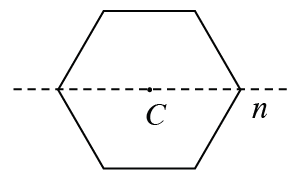### Home > INT1 > Chapter 3 > Lesson 3.1.5 > Problem3-60

3-60.

Read the Math Notes box about polygons in Lesson 3.1.2. At right is a diagram of a regular hexagon with center $C$. Copy this figure on your paper, then complete the problems below.

1. Draw the result of rotating the hexagon about its center $180º$. Explain what happened. When this happens, the polygon has rotation symmetry.

The orientation of the hexagon does not change.

2. What is the result when the original hexagon is reflected across line $n$, as shown at right? A polygon with this quality is said to have reflection symmetry. Line $n$ is called a line of symmetry of the hexagon.

The orientation of the hexagon does not change.

3. Does a regular hexagon have any other lines of symmetry? That is, are there any other lines you could fold across so that both halves of the hexagon will match up? Find as many as you can.